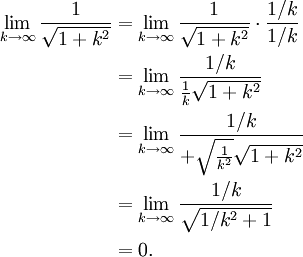# Simple Divergence Test Example

Previous: A Divergence Test Flowchart

Next: Divergence Test With Square Roots

## Problem

Using only the divergence test, determine whether or not the following series diverges## Complete Solution

Applying the divergence test yieldsSince the limit equals zero, the divergence test yields no conclusion.

## Explanation of Each Step

### Step (1)

To apply the divergence test, we replace our sigma with a limit.

To apply our limit, a little algebraic manipulation will help: we may divide both numerator and denominator by the highest power of k that we have. Taking the radical into account, the highest power of k is 1, so we divide both numerator and denominator by k1 = k.

### Step (2)

The algebra in the denominator may be a little tricky. Here is the above derivation with two extra lines of math:## Potential Challenge Areas

### Drawing the Wrong Conclusion

As mentioned earlier, one mistake students could make either one of two mistakes when the limit equals zero:

• that the series converges
• that the convergence of the given series cannot be established

However, when the limit equals zero, the test yields no conclusion, and it could be that the convergence of the given series could be established with a different test. Be careful to not make either of these mistakes.

Previous: A Divergence Test Flowchart

Next: Divergence Test With Square Roots